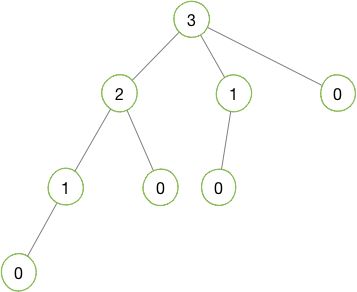## 解题思路

### 问题转换### 答案推导

• $f(1) = 1$
• $f(n) = f(n-1) + f(n-2) + … + f(1) + 1$
• $f(n-1) = \space \space \space \space \space \space \space \space \space \space \space f(n-2) + … + f(1) + 1$

### 最终答案

 1 2 3 4 5 6 7 8  #include class Solution { public: int jumpFloorII(int number) { return pow(2, number - 1); } };